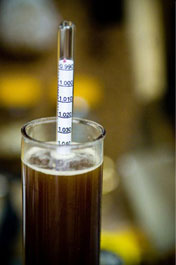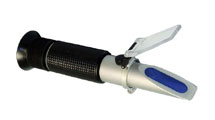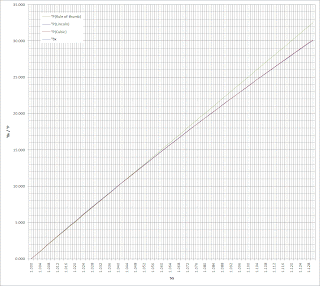## Tuesday, August 17, 2010

### Specific Gravity and Brix/Plato Conversion

If you are buying a new refractometer for brewing purposes, look for one that includes the SG scale (ideally both SG and Brix/Plato) - then you can skip all this conversion nonsense.
I
'm sure a lot of homebrewers face this - you get a new refractometer (which usually measures in degrees Brix or Plato) however almost all homebrewing documentation refers to Specific Gravity (SG). So you go looking for a conversion formula - I mean, how hard could it be? When searching you will find a mix of information: a general "rule of thumb" (see below), a few online calculators (that produce varying results) and a bunch of reference tables - all are workable but the inconsistencies are somewhat disconcerting. I'm the kind of guy that needs to know why things are the way they are, so this blog entry is a summary of what I've found.

#### Specific GravityFirst some background. Specific gravity, or more correctly, relative density, is the ratio of the density of a liquid to the density of pure water.

 SG = DS : DW = DS/DW
Where,
• DS is the density of the sample liquid,
• DW is the density of pure water, and
• SG is the specific gravity.

So, of interest to brewers, when there is more sugar dissolved in a liquid, like unfermented wort, that ratio is greater than after fermentation. The most common tool used by homebrewers to measure the relative density of a liquid is a hydrometer (or more properly called a saccharometer).

#### Temperature Correction

When measuring SG with a hydrometer you should be aware of the temperature of the liquid - especially when dealing with hot wort. Warmer liquid is less dense so the SG will read lower than it would when brought down to room temperature. This is important since hydrometers are calibrated for a specific temperature. The following is a formula for the SG temperature correction for hydrometers calibrated at 15°C (59°F):

 SG' = SG + 1/1000(-2.627634×10-6Ts3 + 2.057793×10-3Ts2 - 1.32674×10-1Ts + 1.313454)
Where,
• SG is the measured specific gravity
• Ts is the temperature of the sample in degrees Fahrenheit (°F), and
• SG' is the corrected specific gravity for a hydrometer calibrated at 59°F.

And of course:

 TF = 9/5TC + 32 ⇔ TC = 5/9(TF - 32)
Where,
• TF is the temperature in degrees Fahrenheit (°F), and
• TC is the temperature in degrees Celsius (°C).

For example, if we have a 40°C (104°F) sample measuring 1.028 in the hydrometer, the formula above tells us that we need to add 0.0068, resulting in a SG of 1.035 - a significant difference.

But not all hydrometers are calibrated for 15°C. So here is a formula for the SG temperature correction for a given hydrometer calibration:

 SG' = SG( 2.32820948×10-9Ts3 + 2.04052596×10-6Ts2 - 1.34722124×10-4Ts + 1.00130346 ) 2.32820948×10-9Tc3 + 2.04052596×10-6Tc2 - 1.34722124×10-4Tc + 1.00130346
Where,
• SG is the measured specific gravity
• Ts is the temperature of the sample in degrees Fahrenheit (°F),
• Tc is the calibration temperature of the hydrometer in degrees Fahrenheit (°F), and
• SG' is the corrected specific gravity.

#### Brewer's Points

Brewers often use a shorthand unit to describe specific gravity called "brewer's points" or just "points". We drop the 1 and multiple by one thousand (moving the decimal point up three spots). For example, an SG of 1.035 is sometimes referred to as 35 points; e.g. 1.035. So mathematically:

 BP = 1000(SG - 1)
Where,
• SG is the specific gravity, and
• BP is the brewer's points.

#### RefractometersSo why use a refractometer? First of all, refractometers are great because you only need a few drops of sample liquid which reduces waste and risk of contamination. Also, most refractometers have an Automatic Temperature Compensation (ATC) so no correction needed. However, a refractometer actually measures the index of refraction of the liquid, not the specific gravity. The good news is that the index of refraction of a liquid is directly related to its density (affected by, for example, the amount of sugar in the liquid), which in turn can be used to calculate its specific gravity.

#### Brix / Plato

The units often used to indicate the amount of sucrose in a liquid are degrees Brix (°Bx) or degrees Plato (°P). There's lots of history as to how these scales were developed and evolved; e.g. the Brix scale is based on the Balling scale (other scales include Baumé and Oechsle). For most purposes, Brix and Plato are interchangeable; e.g. 9.99325°Bx = 9.99359°P. Traditionally brewers use the Plato scale and vintners use the Brix scale. These scales correspond to the percent of sucrose in a pure water solution (by weight). So if we have a 1kg solution that we know contains 90g of sugar (i.e. 910g of water), we would have 9% sugar by weight, that is 9°P, or equivalently 9°Bx.

UPDATE: Most refractometers have been designed for measuring sucrose solutions; unfortunately maltose has a slightly different refractive index (and wort contains some other non-sugar bits that alter the solution's refraction). Every brew is a bit different so one way to estimate the difference is to compare your refractometer with a hydrometer for that particular solution. Typically the refractometer will be somewhere around +2 to +6%. Having to use a hydrometer every time kinda defeats the convenience of a refractometer so most people simply adjust their Brix measurement by 4%.

Bx'  ≈
 Bx 100% + 4%
=
 Bx 1.04
Where,
• Bx is the measured degrees Brix (°Bx) of a maltose solution measured using a sucrose refractometer, and
• Bx' is the adjusted degrees Brix (°Bx).

#### Conversion Formulas

The relationship between SG and the Brix/Plato scales is not linear, it's close but not quite. Several formulas can be used to approximate this curve. For the Plato scale, here are three functions, from the least accurate to the most accurate: a linear equation (Rule of thumb), a quadratic (Lincoln) and a third-order polynomial (Cubic).

PRule of thumb 1000(SG - 1)
4
 BP 4
Where,
• SG is the specific gravity,
• BP is the brewer's points, and
• PRule of thumb is the degrees Plato (°P) calculated using the "Rule of thumb" formula.

 PLincoln = (-205SG + 463)(SG - 1) = -205SG2 + 668SG - 463
Where,
• SG is the specific gravity, and
• PLincoln is the degrees Plato (°P) calculated using the Lincoln quadratic formula.

 PCubic = 135997/1000SG3 - 63034/125SG2 + 11117/50SG - 616217/250 = 135.997SG3 - 630.272SG2 + 1111.14SG - 616.868
Where,
• SG is the specific gravity, and
• PCubic is the degrees Plato (°P) calculated using the cubic formula.

UPDATE: Here is yet another conversion formula associated with the Plato scale:

 PCubic2 = 190.74SG3 - 800.47SG2 + 1286.4SG - 676.67
Where,
• SG is the specific gravity, and
• PCubic2 is the degrees Plato (°P) calculated using another cubic formula.

Here is a conversion formula associated with the Brix scale.

 Bx = 2613/10 ( 1 - 1 ) SG
⇔
 SG = 1 1 - Bx 2613/10
Where,
• SG is the specific gravity, and
• Bx is the degrees Brix (°Bx).Conversion formula comparison
As you can see, the Plato "Rule of thumb" formula is the easiest to remember and is within one SG point up to 15°P. However if you are brewing imperials or other high-gravity beers, you are better off using one of the other formulas (which are all pretty close).

This brings us full circle to the reference tables. Rather than take the time to run the formula, a lookup table is quite handy (although it's good to know which formula the table is based on). Here (TODO) is one that I made and keep with my refractometer. I also use a clear sheet protector to save it from any inadvertent mash splash.

#### Calculators

Here are two calculators that use the formulas described above:

 Brix ⇒ Specific Gravity
Brix (using a sucrose refractomer):   °Bx
()
Adjustment (for a maltose solution):   %
()
Specific gravity (SG):   ⇔   points

 Specific Gravity ⇒ Brix and Plato
Specific gravity (SG):
 ⇔ points () ()
Hydrometer calibration (Tc):
 °C ⇔ °F () ()
Temperature of sample (Ts):
 °C ⇔ °F () ()
Correction (ΔSG):
Corrected specific gravity (SG'):   ⇔   points
Plato: °P(Rule of thumb)
°P(Lincoln)
°P(Cubic)
Brix: °Bx